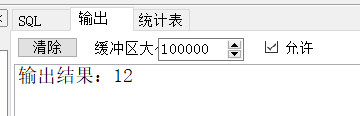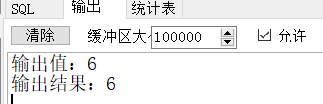• 累计撰写 213 篇文章
• 累计创建 36 个标签
• 累计收到 2 条评论

### 目 录CONTENT# oracle存储过程2021-12-14 / 0 评论 / 0 点赞 / 706 阅读 / 1,402 字 / 正在检测是否收录...

## 存储过程

oracle是存储在数据库的服务端，执行用户的逻辑运算，一次调用后可以反复的在内存中使用

## oracle存储过程的创建语法

create or replace procedure 存储过程名称
(
--定义输入、输出参数--

)
as
--定义变量--
--变量名 变量数据类型；如：
-- numCount integer;
begin
--处理方法-
end;


/*****
** 创建加、减、乘、除计算的存储过程
**输入参数： 数字1，数字2，计算类型
**输出参数： 数字3
*****/
create or replace procedure Proc_Test
(
--定义输入、输出参数--
num_A in integer,
num_B in integer,
numType in integer,
num_C out integer
)
as
--定义变量--
-- numCount integer;
-- numStr varchar(20);
begin
--判断计算类型--
if numType=1 then
num_C := num_A + num_B;
elsif numType=2 then
num_C := num_A - num_B;
elsif numType=3 then
num_C := num_A * num_B;
elsif numType=4 then
num_C := num_A / num_B;
else
--其它处理
dbms_output.put_line('其它处理');
end if;
end;


## 如何调用存储过程

declare num_C integer;
begin
--调用存储过程---
Proc_Test(3,4,3,num_C);
dbms_output.put_line('输出结果：'|| num_C );
end;• 如果我们要在存储过程调用函数，是怎么处理的呢？
create or replace procedure Proc_Test
(
--定义输入、输出参数--
num_A in integer,
num_B in integer,
numType in integer,
num_C out integer
)
as
--定义变量--
-- numStr varchar(20);
begin
/*  --判断计算类型--
if numType=1 then
num_C := num_A + num_B;
elsif numType=2 then
num_C := num_A - num_B;
elsif numType=3 then
num_C := num_A * num_B;
elsif numType=4 then
num_C := num_A / num_B;
else
--其它处理
dbms_output.put_line('其它处理');
end if;*/

--调用函数 并赋值到num_C
num_C:=fun_Test(num_A,num_B,numType);
end;


declare num_C integer;
begin
--调用存储过程---
Proc_Test(12,2,4,num_C);
dbms_output.put_line('输出结果：'|| num_C );
end;0